# 3.2   Binary Search Trees

We examine a symbol-table implementation that combines the flexibility of insertion in linked lists with the efficiency of search in an ordered array. Specifically, using two links per node leads to an efficient symbol-table implementation based on the binary search tree data structure, which qualifies as one of the most fundamental algorithms in computer science.

Definition. A binary search tree (BST) is a binary tree where each node has a Comparable key (and an associated value) and satisfies the restriction that the key in any node is larger than the keys in all nodes in that node's left subtree and smaller than the keys in all nodes in that node's right subtree.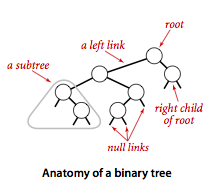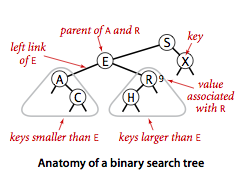## Basic implementation.

Program BST.java implements the ordered symbol-table API using a binary search tree. We define a inner private class to define nodes in BST. Each node contains a key, a value, a left link, a right link, and a node count. The left link points to a BST for items with smaller keys, and the right link points to a BST for items with larger keys. The instance variable N gives the node count in the subtree rooted at the node. This field facilitates the implementation of various ordered symbol-table operations, as you will see.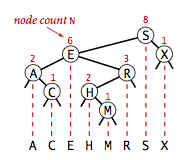• Search. A recursive algorithm to search for a key in a BST follows immediately from the recursive structure: If the tree is empty, we have a search miss; if the search key is equal to the key at the root, we have a search hit. Otherwise, we search (recursively) in the appropriate subtree. The recursive get() method implements this algorithm directly. It takes a node (root of a subtree) as first argument and a key as second argument, starting with the root of the tree and the search key.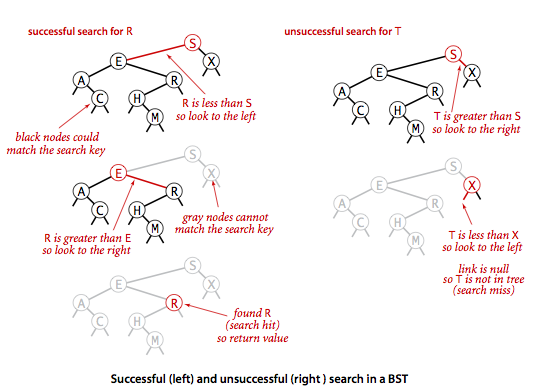• Insert. Insert is not much more difficult to implement than search. Indeed, a search for a key not in the tree ends at a null link, and all that we need to do is replace that link with a new node containing the key. The recursive put() method accomplishes this task using logic similar to that we used for the recursive search: If the tree is empty, we return a new node containing the key and value; if the search key is less than the key at the root, we set the left link to the result of inserting the key into the left subtree; otherwise, we set the right link to the result of inserting the key into the right subtree.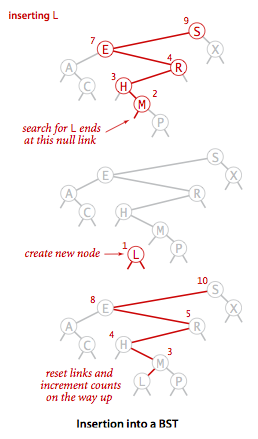## Analysis.

The running times of algorithms on binary search trees depend on the shapes of the trees, which, in turn, depends on the order in which keys are inserted.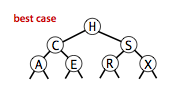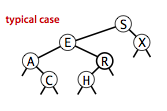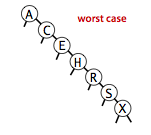It is reasonable, for many applications, to use the following simple model: We assume that the keys are (uniformly) random, or, equivalently, that they are inserted in random order.

### Proposition.

Search hits in a BST built from N random keys requires ~ 2 ln N (about 1.39 lg N) compares on the average.

### Proposition.

Insertion and search misses in a BST built from N random keys requires ~ 2 ln N (about 1.39 lg N) compares on the average.

The visualization below shows the result of inserting 255 keys in a BST in random order. It displays the number of keys (N), the maximum number of nodes on a path from the root to a leaf (max), the average number of nodes on a path from the root to a leaf (avg), the average number of nodes on a path from the root to a leaf in a perfectly balanced BST (opt).

## Order-based methods and deletion.

An important reason that BSTs are widely used is that they allow us to keep the keys in order. As such, they can serve as the basis for implementing the numerous methods in our ordered symbol-table API.

• Minimum and maximum. If the left link of the root is null, the smallest key in a BST is the key at the root; if the left link is not null, the smallest key in the BST is the smallest key in the subtree rooted at the node referenced by the left link. Finding the maximum key is similar, moving to the right instead of to the left.

• Floor and ceiling. If a given key key is less than the key at the root of a BST, then the floor of key (the largest key in the BST less than or equal to key) must be in the left subtree. If key is greater than the key at the root, then the floor of key could be in the right subtree, but only if there is a key smaller than or equal to key in the right subtree; if not (or if key is equal to the key at the root) then the key at the root is the floor of key. Finding the ceiling is similar, interchanging right and left.

• Selection. Suppose that we seek the key of rank k (the key such that precisely k other keys in the BST are smaller). If the number of keys t in the left subtree is larger than k, we look (recursively) for the key of rank k in the left subtree; if t is equal to k, we return the key at the root; and if t is smaller than k, we look (recursively) for the key of rank k - t - 1 in the right subtree.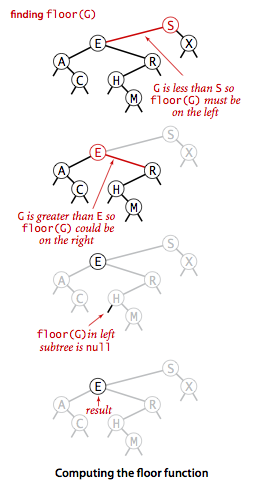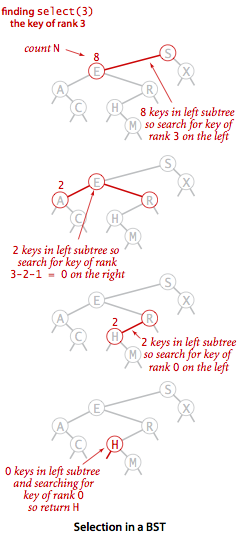• Rank. If the given key is equal to the key at the root, we return the number of keys t in the left subtree; if the given key is less than the key at the root, we return the rank of the key in the left subtree; and if the given key is larger than the key at the root, we return t plus one (to count the key at the root) plus the rank of the key in the right subtree.

• Delete the minimum and maximum. For delete the minimum, we go left until finding a node that that has a null left link and then replace the link to that node by its right link. The symmetric method works for delete the maximum.

• Delete. We can proceed in a similar manner to delete any node that has one child (or no children), but what can we do to delete a node that has two children? We are left with two links, but have a place in the parent node for only one of them. An answer to this dilemma, first proposed by T. Hibbard in 1962, is to delete a node x by replacing it with its successor. Because x has a right child, its successor is the node with the smallest key in its right subtree. The replacement preserves order in the tree because there are no keys between x.key and the successor's key. We accomplish the task of replacing x by its successor in four (!) easy steps:

• Save a link to the node to be deleted in t

• Set x to point to its successor min(t.right).

• Set the right link of x (which is supposed to point to the BST containing all the keys larger than x.key) to deleteMin(t.right), the link to the BST containing all the keys that are larger than x.key after the deletion.

• Set the left link of x (which was null) to t.left (all the keys that are less than both the deleted key and its successor).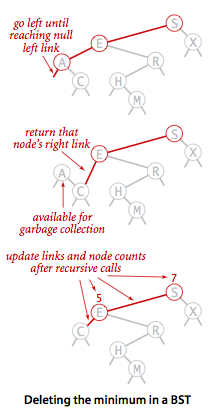While this method does the job, it has a flaw that might cause performance problems in some practical situations. The problem is that the choice of using the successor is arbitrary and not symmetric. Why not use the predecessor?

Each BST contains 150 nodes. We then repeatedly delete (via Hibbard deletion) and insert keys at random. The BST becomes skewed toward the left.

• Range search. To implement the keys() method that returns the keys in a given range, we begin with a basic recursive BST traversal method, known as inorder traversal. To illustrate the method, we consider the task of printing all the keys in a BST in order. To do so, print all the keys in the left subtree (which are less than the key at the root by definition of BSTs), then print the key at the root, then print all the keys in the right subtree, (which are greater than the key at the root by definition of BSTs).
 private void print(Node x) { if (x == null) return; print(x.left); StdOut.println(x.key); print(x.right); } 

To implement the two-argument keys() method, we modify this code to add each key that is in the range to a Queue, and to skip the recursive calls for subtrees that cannot contain keys in the range.

### Proposition.

Search, insertion, finding the minimum, finding the maximum, floor, ceiling, rank, select, delete the minimum, delete the maximum, delete, and range count operations all take time proportional to the height of the tree, in the worst case.

#### Exercises

1. Give five orderings of the keys A X C S E R H that, when inserted into an initially empty BST, produce the best-case tree.

Solution. Any sequence that inserts H first; C before A and E; S before R and X.

2. Add to BST.java a method height() that computes the height of the tree. Develop two implementations: a recursive method (which takes linear time and space proportional to the height), and method like size() that adds a field to each node in the tree (and takes linear space and constant time per query).
3. Write a test client TestBST.java for use in testing the implementations of min(), max(), floor(), ceiling(), select(), rank(), deleteMin(), deleteMax(), and keys() that are given in the text.
4. Give nonrecursive implementations of get(), put(), and keys() for BST.

Solution: NonrecursiveBST.java

#### Creative Problems

1. Perfect balance. Write a program PerfectBalance.java that inserts a set of keys into an initially empty BST such that the tree produced is equivalent to binary search, in the sense that the sequence of compares done in the search for any key in the BST is the same as the sequence of compares used by binary search for the same set of keys.

Hint: Put the median at the root and recursively build the left and right subtree.

2. Certification. Write a method isBST() in BST.java that takes a Node as argument and returns true if the argument node is the root of a binary search tree, false otherwise.
3. Subtree count check. Write a recursive method isSizeConsistent() in BST.java that takes a Node as argument and returns true if the subtree count field N is consistent in the data structure rooted at that node, false otherwise.
4. Select/rank check. Write a method isRankConsistent() in BST.java that checks, for all i from 0 to size() - 1, whether i is equal to rank(select(i)) and, for all keys in the BST, whether key is equal to select(rank(key)).

#### Web Exercises

1. The great tree-list recursion problem. A binary search tree and a circular doubly linked list are conceptually built from the same type of nodes - a data field and two references to other nodes. Given a binary search tree, rearrange the references so that it becomes a circular doubly-linked list (in sorted order). Nick Parlante describes this as one of the neatest recursive pointer problems ever devised. Hint: create a circularly linked list A from the left subtree, a circularly linked list B from the right subtree, and make the root a one node circularly linked list. Them merge the three lists.
2. BST reconstruction. Given the preorder traversal of a BST (not including null nodes), reconstruct the tree.
3. True or false. Given a BST, let x be a leaf node, and let y be its parent. Then either (i) the key of y is the smallest key in the BST larger than the key of x or (ii) the key of y is the largest key in the BST smaller than the key of x. Answer: true.
4. True or false. Let x be a BST node. The next largest key (successor of x) can be found by traversing up the tree toward the root until encountering a node with a non-empty right subtree (possibly x itself); then finding the minimum key in the right subtree (by following its rightmost path).
5. Tree traversal with constant extra memory. Describe how to perform an inorder tree traversal with constant extra memory (e.g., no function call stack).

Hint: on the way down the tree, make the child node point back to the parent (and reverse it on the way up the tree).

6. Reverse a BST. Given a standard BST (where each key is greater than the keys in its left subtree and smaller than the keys in its right subtree), design a linear-time algorithm to transform it into a reverese BST (where each key is smaller than the keys in its left subtree and greater than the keys in its right subtree). The resulting tree shape should be symmetric to the original one.
7. Level-order traversal reconstruction of a BST. Given a sequence of keys, design a linear-time algorithm to determine whether it is the level-order traversal of some BST (and construct the BST itself).
8. Find two swapped keys in a BST. Given a BST in which two keys in two nodes have been swapped, find the two keys.

Solution. Consider the inorder traversal a[] of the BST. There are two cases to consider. Suppose there is only one index p such that a[p] > a[p+1]. Then swap the keys a[p] and a[p+1]. Otherwise, there are two indices p and q such a[p] > a[p+1] and a[q] > a[q+1]. Let's assume p < q. Then, swap the keys a[p] and a[q+1].